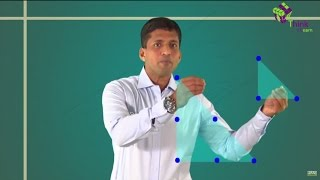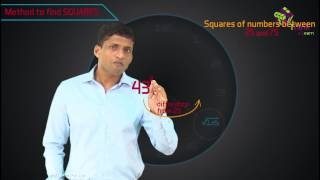# Maths#### Square of a NumberLEARNING MATHS WITH FUN

Maths is a subject which is not only applicable in our academics but also in real life. Making kids love this subject is a challenge, especially for parents. It takes a lot of brainpower to master Maths and this can be tough for kids. Thus, BYJU’S presents here a simple and easy way for kids and higher class students to learn Maths, with more interest.

Online Maths for free has been brought here for the ease of students, so that they can get access to each and every fundamental concept and learn quickly. Practice Maths here with the given examples and practice questions for all the Class from 1 to 12. For better practice, worksheets are also provided by us, so that students can excel the concepts.

## Maths Mapping

In mapping Maths, we will come across many concepts. The origin or base of Maths is Counting, where we learned counting the objects that are visible to our eye. Mathematics are broadly classified into two groups: Pure Mathematics (number system, geometry, matrix, algebra, combinatorics, topology, calculus) and Applied Mathematics (Engineering, Chemistry, Physics, numerical analysis, etc).

Maths has a lot of formulas based on different concepts. These formulas can be memorized by practising questions based on them. Some problems can be solved quickly, using Maths tricks. Class 1 to 10 has been taught with the general mathematical concepts, but it’s level increases in Class 11 and 12.

## Maths Online

At the starting level, basics of Math have been taught such as counting the numbers, addition, subtraction, multiplication, division, place value, etc. As the level of grade increases, students are taught with more enhanced concepts, such as ratios, proportions, fractions, algebra, geometry, trigonometry, mensuration, etc. Integration and differentiation are the higher level of topics, which are included in the syllabus of higher secondary school. Get Math syllabus for class 9 to 12, here and prepare your studies.

## How to Learn Maths?

Maths is a fun subject. But learning Maths has never been easy. At BYJU’S, you can create such a strong foundation in this subject, with the help of which any mathematical problems would seem to be a cakewalk to you. Getting a good grip in Math fundamentals, through understanding and practice, will tend to develop your brain in a logical way and help you to grasp the concepts in all other subjects with greater speed and efficiency.

We are providing learning materials for students of Class 6 to 12 based on the syllabus. In these classes, students set their foot into the world of all the important theories in Maths, like whole numbers, basic geometry, integers, decimals, algebra, ratio and proportion, etc. Since kids are more receptive to learning, when it is associated more with play than with work, hence it is a good idea for parents to expose kids to math in fun ways. We provide lessons for students till class 12, where they study the most complex topics like 3-D geometry, vector algebra, differential equations, matrices, etc. A few of the links of important topics have been shared here for your convenience.

With the help of animations and innovative ways of teaching by our experts, the ideas and concepts are sure to stay etched in your minds forever.  The purpose is to make math fun and help kids grow to understand that math is fun. Instead of being afraid, the kids will then be excited about the subject for the rest of their educational journey. You can engage yourself with interactive video sessions, regular tests prepared by our experts and do continuous analysis based on your performance.

The interactive video lessons on BYJU’S learning app will give you very elaborate and detailed explanations of the various ideas in Maths.

Online Tools & Calculators

Inches To Cm Converter

Cm To Inches Converter

## Basic Maths

The basics of Maths deals with simple arithmetic operations, which are:

• Subtraction (-)
• Multiplication (x)
• Division (÷)
• To become an expert in these basic concepts, students need to practise questions and solve worksheets based on them. There will few more basics such as multiples, factors, HCF and LCM, which will be introduced to the students in their secondary classes.

Multiplication tables are also one of the fundamentals of Maths. Students will have to learn tables from 1 to 10 in their primary school to solve multiplication problems.

### What are the different branches of maths?

The major branches of maths are:

• Number system and basic Arithmetic
• Algebra
• Trigonometry
• Geometry and Cartesian Geometry
• Calculus- Differential and Integral
• Matrix Algebra
• Probability and Statistics

### What are the fundamentals of Maths?

Fundamentals of Maths covers basic arithmetic operations or calculations such as addition, subtraction, multiplication and division, which are taught to us in primary classes. Going forward, in higher classes, students will learn basic concepts like algebra, geometry, factors, ratios, etc.

### What are the different maths resources available at BYJU’S?

BYJU’S provides a lot of maths resources along with the conceptual explanations. Some of the resources that students can access for free at BYJU’S are:

• NCERT, RS Aggarwal and RD Sharma Solutions
• CBSE sample papers and question papers
• Video lessons
• Maths worksheets
• Lessons in PDF format
• Syllabus and exam blueprints
• Important questions for board exams
• Revision notes

### What are the basics of Geometry?

In geometry, we basically learn about different shapes and sizes in two-dimension and three-dimension. But we begin with point, lines and angles which are base of geometry.
Circle, Square, Rectangle, Parallelogram, Rhombus, Kite, etc. are two-dimensional shapes.
Cube, Cuboid, Sphere, Cylinder, Cone are the three-dimensional shapes.

### What are the basic arithmetic operations?

The four basic maths operations are:
Subtraction
Multiplication
Division

1. vivek ns

hello
what are the products available for mathematics and physics

1. Charani

Divisions sir

2. Byju is good

2. Franklin Bouba

Hello thank you for you service

3. byjus app excellent education app

4. praveen

have a good bijus app to understand lessons easily

5. kamlesh kumar

Hi sir mujhe ssc cgl math ka solution chahiye

6. Olawale

Hello thanks for ur service

1. Dj alok

7. fjthju

I am looking for a topic on angles grade 6

8. Kislay kumar

Hay sir kislay kumar 9th ke math ka solution chahta hu

9. Amy Jacob

Thankyou Byjus app
To make me interesting in mathematics and for videos

10. Hii nice class we understand easily and good keep it up

11. Vernika Singh

Sir I need fundamental concepts solutions of class 8.

12. vineet kumar

Sir my buyju’s app not responding, so sir you help me.

13. Dali Dersak

Can I take only math from byju’s app ? How much is the charges ? My son is in 7 th grade . I am from Sharjah

1. can i take only math from byjus app? how much is the charges , iam tryingt study for jee 2021

14. Harsh Lamba

15. Jagriti Patel

Mam l am grad 7 students mam please send my syllabus and geometry questions

16. But I am happy with byju

17. kirabo esther

Thanks for service

18. Rishabh kumar

byjus is very good app

19. Nirjara Pani

It is a nice app to learn at home.

20. ANSHIKA PANDEY

It is very good app to learn at home

21. Farisha

Good understanding

22. k vinay sai Sandeep kumar

Byju’s is good and help in study’s